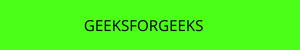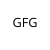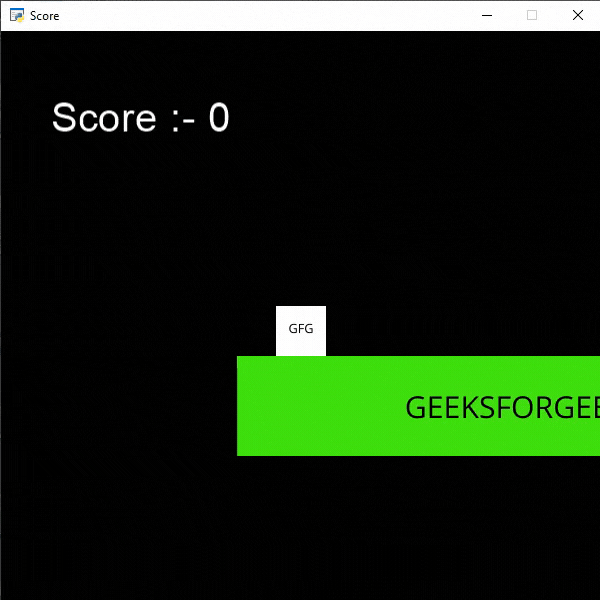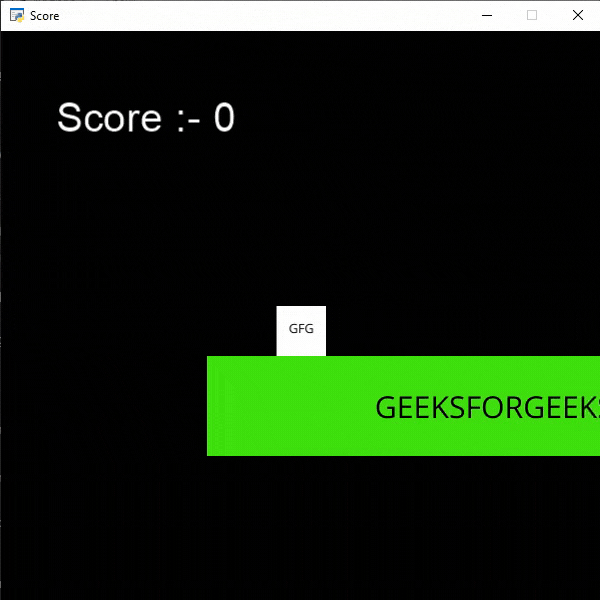# Python Arcade – Display Score

• Difficulty Level : Expert
• Last Updated : 23 Sep, 2021

## Displaying the Score

In this example, we are going to simply display our score on the screen and we will increase the score every player jumps. For this, we will use some functions:

Attention geek! Strengthen your foundations with the Python Programming Foundation Course and learn the basics.

To begin with, your interview preparations Enhance your Data Structures concepts with the Python DS Course. And to begin with your Machine Learning Journey, join the Machine Learning - Basic Level Course

draw_text(): This function is used to draw text to the screen using Pyglet’s label.

Syntax: arcade.draw_text(text, x, y, color, size, width, align, font_name)

Parameters:

• text: Text we want to display
• x : x coordinate
• y : y coordinate
• color : color of the text
• size : Size of the font
• width : Width of the text
• align : Alignment of the text
• font_name : Name of the font

Camera(): The Camera class is used for controlling the visible viewport.

Syntax: arcade.Camera( width , height, window)

Parameters:

• width: width of the viewport
• height: height of the viewport
• window: Window to associate with this camera

Scene(): A class that represents a scene object.

Parameters:

• sprite_lists: A list of SpriteList objects
• name_mapping: A dictionary of SpriteList objects

PhysicsEnginePlatformer(): Simplistic physics engine for use in a platformer.

Parameters:

• player_sprite: sprite of the player
• platforms: The sprites it can’t move through
• gravity: Downward acceleration per frame

Sprites Used():In the below example, we are going to create a MainGame() class. Inside this class first, we are going to initialize some variables for velocity, camera, score, level, and player’s sprite then we will create 6 functions inside this class.

• on_draw(): Inside this function, we will use our camera and draw the scene and our score text on the screen.
• setup(): In this function, we will initialize our camera and scene object then we will load our player and platform’s sprites. After that, we will call the  PhysicsEnginePlatformer() function.
• on_update(): In this function, we will update the x coordinates of the player’s sprite, camera, and physics engine. We will also change our level in this function.
• on_key_press() and on_key_release(): In this function, we will change the value of the velocity variable according to the keyboard key that is pressed or released. And if the player presses the UP arrow key then we will increase the value of the score.
• camera_move(): In this function, we will move our camera according to our player’s current position.

Below is the implementation:

## Python3

 `# Importing arcade module``import` `arcade`` ` `# Creating MainGame class``class` `MainGame(arcade.Window):``    ``def` `__init__(``self``):``        ``super``().__init__(``600``, ``600``,``                         ``title``=``"Player Movement"``)`` ` `        ``# Initializing a variable to store``        ``# the velocity of the player``        ``self``.vel_x ``=` `0`` ` `        ``# Creating variable for Camera``        ``self``.camera ``=` `None`` ` `        ``# Creating variable to store current score``        ``self``.score ``=` `0`` ` `        ``# Creating scene object``        ``self``.scene ``=` `None`` ` `        ``# Creating variable to store player sprite``        ``self``.player ``=` `None`` ` `        ``# Creating variable for our game engine``        ``self``.physics_engine ``=` `None`` ` `    ``# Creating on_draw() function to draw on the screen``    ``def` `on_draw(``self``):``        ``arcade.start_render()`` ` `        ``# Drawing the text``        ``arcade.draw_text(``'Score :- '``+``str``(``self``.score), ``                         ``self``.player_sprite.center_x ``-` `500` `/` `2``,``                         ``self``.player_sprite.center_y ``+` `200``,``                         ``arcade.color.WHITE, ``30``, ``5000``, ``'left'``)`` ` `        ``# Using the camera``        ``self``.camera.use()``        ``# Drawing our scene``        ``self``.scene.draw()`` ` `    ``def` `setup(``self``):``       ` `         ``# Initialize Scene object``        ``self``.scene ``=` `arcade.Scene()\``         ` `        ``# Using Camera() function``        ``self``.camera ``=` `arcade.Camera(``600``, ``600``)`` ` `        ``# Creating different sprite lists``        ``self``.scene.add_sprite_list(``"Player"``)``        ``self``.scene.add_sprite_list(``"Platforms"``,``                                   ``use_spatial_hash``=``True``)`` ` `        ``# Adding player sprite``        ``self``.player_sprite ``=` `arcade.Sprite(``"Player.png"``, ``1``)`` ` `        ``# Adding coordinates for the center of the sprite``        ``self``.player_sprite.center_x ``=` `64``        ``self``.player_sprite.center_y ``=` `60`` ` `        ``# Adding Sprite in our scene``        ``self``.scene.add_sprite(``"Player"``, ``self``.player_sprite)`` ` `        ``# Adding platform sprite according to level``        ``platform ``=` `arcade.Sprite(f``"Platform.png"``, ``1``)``         ` `        ``# Adding coordinates for the center of the platform``        ``platform.center_x ``=` `300``        ``platform.center_y ``=` `32``        ``self``.scene.add_sprite(``"Platforms"``, platform)`` ` `        ``# Creating Physics engine``        ``self``.physics_engine ``=` `arcade.PhysicsEnginePlatformer(``            ``self``.player_sprite, ``self``.scene.get_sprite_list(``"Platforms"``), ``0.5``        ``)`` ` `    ``# Creating on_update function to``    ``# update the x coordinate``    ``def` `on_update(``self``, delta_time):`` ` `        ``# Changing x coordinate of player``        ``self``.player_sprite.center_x ``+``=` `self``.vel_x ``*` `delta_time``         ` `        ``# Updating the physics engine to move the player``        ``self``.physics_engine.update()`` ` `        ``# Calling the camera_move function``        ``self``.camera_move()`` ` `    ``# Creating function to change the velocity``    ``# when button is pressed`` ` `    ``def` `on_key_press(``self``, symbol, modifier):`` ` `        ``# Checking the button pressed``        ``# and changing the value of velocity``        ``if` `symbol ``=``=` `arcade.key.LEFT:``            ``self``.vel_x ``=` `-``300``        ``elif` `symbol ``=``=` `arcade.key.RIGHT:``            ``self``.vel_x ``=` `300``        ``elif` `symbol ``=``=` `arcade.key.UP:``            ``if` `self``.physics_engine.can_jump():``                ``self``.player_sprite.change_y ``=` `15``                 ` `                ``# Increasing the score``                ``self``.score ``+``=` `10`` ` `    ``# Creating function to change the velocity``    ``# when button is released`` ` `    ``def` `on_key_release(``self``, symbol, modifier):`` ` `        ``# Checking the button released``        ``# and changing the value of velocity``        ``if` `symbol ``=``=` `arcade.key.LEFT:``            ``self``.vel_x ``=` `0``        ``elif` `symbol ``=``=` `arcade.key.RIGHT:``            ``self``.vel_x ``=` `0`` ` `    ``def` `camera_move(``self``):`` ` `        ``# Getting the x coordinate for the center of camera``        ``screen_x ``=` `self``.player_sprite.center_x ``-` `\``            ``(``self``.camera.viewport_width ``/` `2``)`` ` `        ``# Getting the y coordinate for the center of camera``        ``screen_y ``=` `self``.player_sprite.center_y ``-` `\``            ``(``self``.camera.viewport_height ``/` `2``)`` ` `        ``# Moving the camera``        ``self``.camera.move_to([screen_x, screen_y])`` ` `# Calling MainGame class``game ``=` `MainGame()``game.setup()``arcade.run()`

Output:## Resetting the Score

In this example, we will reset our score to 0 whenever the player falls from the platform and restart the game from starting position. For this, we will simply add the below lines in our on_update() function.

```if self.player_sprite.center_y < -20:
self.score = 0
self.setup()```

If the player falls from the platform then ware setting the value of the score variable to 0 and calling our setup() function to restart the game.

Below is the implementation:

## Python3

 `# Importing arcade module``import` `arcade`` ` `# Creating MainGame class``class` `MainGame(arcade.Window):``    ``def` `__init__(``self``):``        ``super``().__init__(``600``, ``600``,``                         ``title ``=` `"Score"``)`` ` `        ``# Initializing a variable to store``        ``# the velocity of the player``        ``self``.vel_x ``=` `0`` ` `        ``# Creating variable for Camera``        ``self``.camera ``=` `None`` ` `        ``# Creating variable to store current score``        ``self``.score ``=` `0`` ` `        ``# Creating scene object``        ``self``.scene ``=` `None`` ` `        ``# Creating variable to store player sprite``        ``self``.player ``=` `None`` ` `        ``# Creating variable for our game engine``        ``self``.physics_engine ``=` `None`` ` `    ``# Creating on_draw() function to draw on the screen``    ``def` `on_draw(``self``):``        ``arcade.start_render()`` ` `        ``# Drawing the text``        ``arcade.draw_text(``'Score :- '``+``str``(``self``.score),``                         ``self``.player_sprite.center_x ``-``500` `/` `2``,``                         ``self``.player_sprite.center_y ``+``200``,``                         ``arcade.color.WHITE,``30``,``5000``,``'left'``)`` ` `        ``# Using the camera``        ``self``.camera.use()``        ``# Drawing our scene``        ``self``.scene.draw()`` ` `    ``def` `setup(``self``):``       ` `         ``# Initialize Scene object``        ``self``.scene ``=` `arcade.Scene()``         ` `        ``# Using Camera() function``        ``self``.camera ``=` `arcade.Camera(``600``, ``600``)`` ` `        ``# Creating different sprite lists``        ``self``.scene.add_sprite_list(``"Player"``)``        ``self``.scene.add_sprite_list(``"Platforms"``,``                                   ``use_spatial_hash``=``True``)`` ` `        ``# Adding player sprite``        ``self``.player_sprite ``=` `arcade.Sprite(``"Player.png"``, ``1``)`` ` `        ``# Adding coordinates for the center of the sprite``        ``self``.player_sprite.center_x ``=` `64``        ``self``.player_sprite.center_y ``=` `60`` ` `        ``# Adding Sprite in our scene``        ``self``.scene.add_sprite(``"Player"``, ``self``.player_sprite)`` ` `        ``# Adding platform sprite according to level``        ``platform ``=` `arcade.Sprite(f``"Platform.png"``, ``1``)``         ` `        ``# Adding coordinates for the center of the platform``        ``platform.center_x ``=` `300``        ``platform.center_y ``=` `32``        ``self``.scene.add_sprite(``"Platforms"``, platform)`` ` `        ``# Creating Physics engine``        ``self``.physics_engine ``=` `arcade.PhysicsEnginePlatformer(``            ``self``.player_sprite, ``self``.scene.get_sprite_list(``"Platforms"``), ``0.5``        ``)`` ` `    ``# Creating on_update function to``    ``# update the x coordinate``    ``def` `on_update(``self``, delta_time):`` ` `        ``# Changing x coordinate of player``        ``self``.player_sprite.center_x ``+``=` `self``.vel_x ``*` `delta_time``         ` `        ``# Updating the physics engine to move the player``        ``self``.physics_engine.update()`` ` `        ``# Setting the score to 0 whenever``        ``# player falss from the platform``        ``if` `self``.player_sprite.center_y < ``-``20``:``            ``self``.score ``=` `0``            ``self``.setup()`` ` `        ``# Calling the camera_move function``        ``self``.camera_move()``         ` `    ``# Creating function to change the velocity``    ``# when button is pressed``    ``def` `on_key_press(``self``, symbol, modifier):`` ` `        ``# Checking the button pressed``        ``# and changing the value of velocity``        ``if` `symbol ``=``=` `arcade.key.LEFT:``            ``self``.vel_x ``=` `-``300``        ``elif` `symbol ``=``=` `arcade.key.RIGHT:``            ``self``.vel_x ``=` `300``        ``elif` `symbol ``=``=` `arcade.key.UP:``            ``if` `self``.physics_engine.can_jump():``                ``self``.player_sprite.change_y ``=` `15``                 ` `                ``# Increasing the score``                ``self``.score ``+``=` `10`` ` ` ` `    ``# Creating function to change the velocity``    ``# when button is released``    ``def` `on_key_release(``self``, symbol, modifier):`` ` `        ``# Checking the button released``        ``# and changing the value of velocity``        ``if` `symbol ``=``=` `arcade.key.LEFT:``            ``self``.vel_x ``=` `0``        ``elif` `symbol ``=``=` `arcade.key.RIGHT:``            ``self``.vel_x ``=` `0`` ` `    ``def` `camera_move(``self``):``       ` `        ``# Getting the x coordinate for the center of camera``        ``screen_x ``=` `self``.player_sprite.center_x ``-` `\``            ``(``self``.camera.viewport_width ``/` `2``)``         ` `        ``# Getting the y coordinate for the center of camera``        ``screen_y ``=` `self``.player_sprite.center_y ``-` `\``            ``(``self``.camera.viewport_height ``/` `2``)`` ` `        ``# Moving the camera``        ``self``.camera.move_to([screen_x, screen_y])`` ` `# Calling MainGame class``game ``=` `MainGame()``game.setup()``arcade.run()`

Output:My Personal Notes arrow_drop_up